0
231

# Geometry Circle Questions for SSC MTS

Here you can download the Geometry Circle Questions for SSC MTS PDF with solutions by Cracku. These are the most important Geometry Circle questions PDF prepared by various sources also based on previous year’s papers. Utilize this PDF for Geometry Circle for SSC MTS preparation. You can find a list of the most important Geometry Circle questions in this PDF which help you to test yourself and practice. So you can click on the below link to download the PDF for reference and do more practice.

Question 1: In a circle with centre O, points A, B, C and D in this order are concyclic such that BD is a diameter of the circle. If $\angle$BAC = 22$^\circ$, then find the measure (in degrees) of $\angle$COD.

a) 79

b) 136

c) 158

d) 68

Solution: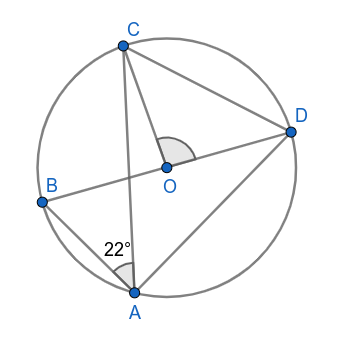Angle in a semicircle is right angle.

$\Rightarrow$  $\angle$BAD = 90$^\circ$

$\Rightarrow$  $\angle$BAC + $\angle$CAD = 90$^\circ$

$\Rightarrow$  22$^\circ$ + $\angle$CAD = 90$^\circ$

$\Rightarrow$  $\angle$CAD = 68$^\circ$

Angle subtended by a chord at the center of the circle is twice the angle subtended by the chord on the point of a circle in the same segment.

$\Rightarrow$  $\angle$COD = 2$\angle$CAD

$\Rightarrow$   $\angle$COD = 136$^\circ$

Hence, the correct answer is Option B

Question 2: Vertices A, B, C and D of a quadrilateral ABCD lie on a circle. $\angle$A is three times $\angle$C and $\angle$D is two times $\angle$B. What is the difference between the measures of $\angle$D and $\angle$C?

a) $55^\circ$

b) $65^\circ$

c) $75^\circ$

d) $45^\circ$

Solution: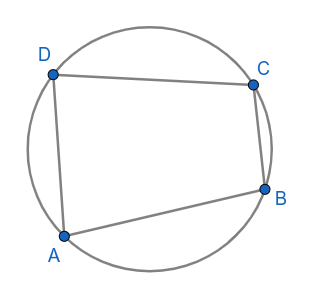Given,

$\angle$A is three times $\angle$C and $\angle$D is two times $\angle$B.

$\angle$A = 3$\angle$C…….(1)

$\angle$D = 2$\angle$B…….(2)

In a cyclic quadrilateral, opposite angles are supplementary.

$\angle$A + $\angle$C = 180$^\circ$ and $\angle$B + $\angle$D = 180$^\circ$

$\angle$A + $\angle$C = 180$^\circ$

3$\angle$C + $\angle$C = 180$^\circ$  [From (1)]

4$\angle$C = 180$^\circ$

$\angle$C = 45$^\circ$

$\angle$A = 3$\angle$C = 135$^\circ$

$\angle$B + $\angle$D = 180$^\circ$

$\angle$B + 2$\angle$B = 180$^\circ$  [From (2)]

3$\angle$B = 180$^\circ$

$\angle$B = 60$^\circ$

$\angle$D = 2$\angle$B = 120$^\circ$

Difference between the measures of $\angle$D and $\angle$C = 120$^\circ$ – 45$^\circ$

= 75$^\circ$

Hence, the correct answer is Option C

Question 3: Points A, B and C are on circle with centre O such that $\angle$BOC = 84$^\circ$. If AC is produced to a point D such that $\angle$BDC = 40$^\circ$, then find the measure of $\angle$ABD (in degrees).

a) 98

b) 92

c) 56

d) 102

Solution: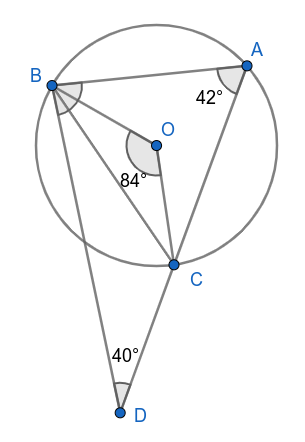Angle subtended by chord BC at the centre is twice the angle subtended by chord BC on the point A of the circle.

$\angle$BOC = 2$\angle$BAC

84$^\circ$ = 2$\angle$BAC

$\angle$BAC = 42$^\circ$

$\angle$BAD + $\angle$ABD + $\angle$BDA = 180$^\circ$

42$^\circ$ + $\angle$ABD + 40$^\circ$ = 180$^\circ$

$\angle$ABD = 98$^\circ$

Hence, the correct answer is Option A

Question 4: A square has the perimeter equal to the circumference of a circle having radius 7 cm. What is the ratio of the area of the circle to area of the square?(Use $\pi = \frac{22}{7}$)

a) 121 : 44

b) 7 : 2

c) 14 : 11

d) 7 : 11

Solution:

Radius of the circle = 7 cm

Circumference of the circle = $2\times\frac{22}{7}\times7$ = 44 cm

Perimeter of square is equal to the circumference of the circle.

Perimeter of the square = 44 cm

Let the side of the square = a

4a = 44

a = 11 cm

Ratio of the area of the circle to area of the square = $\frac{22}{7}\times7^2\ :\ 11^2$

= 14 : 11

Hence, the correct answer is Option C

Question 5: The area of a circular path enclosed by two concentric circles is 3080 m$^2$. If the difference between the radius of the outer edge and that of inner edge of the circular path is 10 m, what is the sum (in m) of the two radii? (Take $\pi = \frac{22}{7}$)

a) 112

b) 70

c) 84

d) 98

Solution:

Let the radius of the outer circle and inner circle are $r_o$ and $r_i$ respectively.

The difference between the radius of the outer edge and that of inner edge of the circular path is 10 m.

$r_o$ – $r_i$ = 10……..(1)

The area of a circular path enclosed by two concentric circles is 3080 m$^2$.

$\Rightarrow$  $\frac{22}{7}r_o^2\ -\frac{22}{7}r_i^2=3080$

$\Rightarrow$  $\left(r_o+r_i\right)\left(r_o-r_i\right)=140\times7$

$\Rightarrow$  $\left(r_o+r_i\right)\left(10\right)=140\times7$

$\Rightarrow$  $\left(r_o+r_i\right)=98$

Sum of the two radii = 98 m

Hence, the correct answer is Option D

Question 6: Tangent is drawn from a point P to a circle, which meets the circle at T such that PT = 8 cm. A secant PAB intersects the circle in points A and B. If PA= 5 cm, what is the length (in cm) of the chord AB?

a) 8.0

b) 8.4

c) 6.4

d) 7.8

Solution: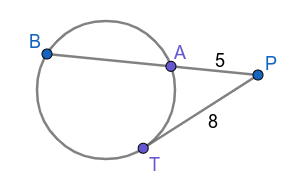$\Rightarrow$  PT$^2$ = PA. PB

$\Rightarrow$  8$^2$ = 5 x (PA + AB)

$\Rightarrow$  64 = 5 x (5 + AB)

$\Rightarrow$  12.8 = 5 + AB

$\Rightarrow$  AB = 7.8 cm

Hence, the correct answer is Option D

Question 7: Two circles of radius 15 cm and 37 cm intersect each other at the points A and B. If the length of common chord is 24 cm, what is the distance (in cm) between the centres of the circles?

a) 44

b) 45

c) 42

d) 40

Solution: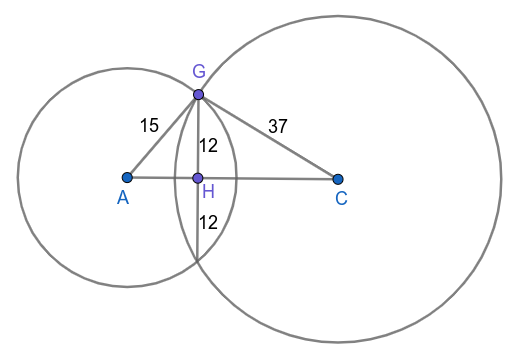From triangle AHG,

AH$^2$ + GH$^2$ = AG$^2$

AH$^2$ + 12$^2$ = 15$^2$

AH$^2$ + 144 = 225

AH$^2$ = 81

AH = 9 cm

From triangle CHG,

CH$^2$ + GH$^2$ = CG$^2$

CH$^2$ + 12$^2$ = 37$^2$

CH$^2$ + 144 = 1369

CH$^2$ = 1225

CH = 35 cm

Distance between two circles = AC = AH + CH = 9 + 35 = 44 cm

Hence, the correct answer is Option A

Question 8: Two circles of radii 18 cm and 16 cm intersect each other and the length of their common chord is 20 cm. What is the distance (in cm) between their centres?

a) $4\sqrt{14} – 2\sqrt{39}$

b) $4\sqrt{10} – 2\sqrt{39}$

c) $4\sqrt{10} + 2\sqrt{39}$

d) $4\sqrt{14} + 2\sqrt{39}$

Solution: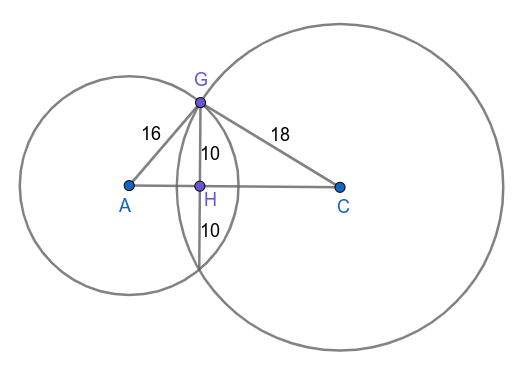From triangle AGH,

AH$^2$ + GH$^2$ = AG$^2$

AH$^2$ + 10$^2$ = 16$^2$

AH$^2$ + 100 = 256

AH$^2$ = 156

AH = $2\sqrt{39}$

From triangle CGH,

CH$^2$ + GH$^2$ = CG$^2$

CH$^2$ + 10$^2$ = 18$^2$

CH$^2$ + 100 = 324

CH$^2$ = 224

CH = $4\sqrt{14}$

Distance between centres of circles = AC = AH + CH = $2\sqrt{39}$ + $4\sqrt{14}$

Hence, the correct answer is Option D

Question 9: The area of a quadrant of a circle is $\frac{\pi}{9}$ m$^2$. Its radius (in metres) is equal to:

a) $\frac{2}{3}$

b) $\frac{1}{3}$

c) $\frac{1}{2}$

d) $\frac{3}{2}$

Solution:

Let the radius of the circle = r

Area of the quadrant of the circle = $\frac{\pi}{9}$ m$^2$

$\frac{1}{4}\times\pi$r$^2$ = $\frac{\pi}{9}$

r$^2$ = $\frac{4}{9}$

r = $\frac{2}{3}$

Radius of the circle = $\frac{2}{3}$ m

Hence, the correct answer is Option A

Question 10: In a circle with centre O, AB and CD are parallel chords on the opposite sides of a diameter. If AB = 12 cm, CD = 18 cm and the distance between the chords AB and CD is 15 cm, then find the radius of the circle (in cm).

a) $9\sqrt{13}$

b) 9

c) $3\sqrt{13}$

d) 12

Solution: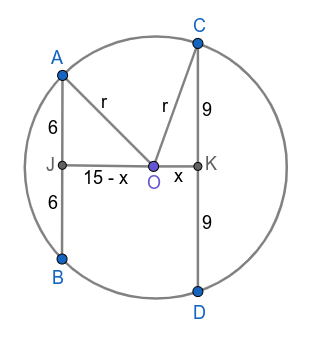From triangle AJO,

r$^2$ = 6$^2$ + (15 – x)$^2$

r$^2$ = 36 + (15 – x)$^2$………………(1)

From triangle CKO,

r$^2$ = 9$^2$ + x$^2$

r$^2$ = 81 + x$^2$………………(2)

From (1) and (2),

36 + (15 – x)$^2$ = 81 + x$^2$

225 + x$^2$ – 30x = 45 + x$^2$

30x = 180

x = 6

From (2),

r$^2$ = 81 + x$^2$

r$^2$ = 81 + 6$^2$

r$^2$ = 81 + 36

r$^2$ = 137

r = $3\sqrt{13}$

Hence, the correct answer is Option C

Question 11: In the figure, a circle touches all the four sides of a quadrilateral ABCD whose sides AB = 6.5 cm, BC = 5.4 cm and CD = 5.3 cm. The length of AD is: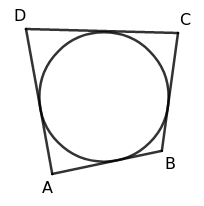a) 4.6 cm

b) 5.8 cm

c) 6.2 cm

d) 6.4 cm

Solution: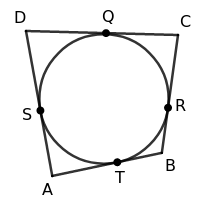Given, AB = 6.5 cm, BC = 5.4 cm and CD = 5.3 cm

Let the circle touches AB, BC, CD, DA at T, R, Q, S respectively.

Length of tangents to the circle from an external point are equal.

AT = AS

BT = BR

CQ = CR

DQ = DS

AT + BT + CQ + DQ = AS + BR + CR + DS

$\Rightarrow$  (AT + BT) + (CQ + DQ) = (AS + DS) + (BR + CR)

$\Rightarrow$  AB + CD = AD + BC

$\Rightarrow$  6.5 + 5.3 = AD + 5.4

$\Rightarrow$  AD = 6.4 cm

Hence, the correct answer is Option D

Question 12: The circles of same radius 13 cm intersect each other at A and B. If AB = 10 cm, then the distance between their centres is:

a) 18 cm

b) 12 cm

c) 24 cm

d) 26 cm

Solution: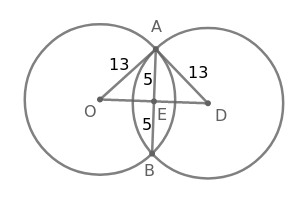Given, AB = 10 cm

AB is the common chord for both the circles and OD will be the perpendicular bisector of AB.

$\Rightarrow$ AE = EB = 5 cm

In $\triangle\$AOE,

OE$^2$ + AE$^2$ = OA$^2$

$\Rightarrow$  OE$^2$ + 5$^2$ = 13$^2$

$\Rightarrow$  OE$^2$ + 25 = 169

$\Rightarrow$  OE$^2$ = 144

$\Rightarrow$  OE = 12 cm

Similarly, in $\triangle$AED

ED = 12 cm

$\therefore\$Distance between the centres of the circles = OD = OE + ED = 12 + 12 = 24 cm

Hence, the correct answer is Option C

Question 13: The area of the quadrant of a circle whose circumference is 22 cm, will be:

a) 3.5 $cm^2$

b) 38.5 $cm^2$

c) 10 $cm^2$

d) 9.625 $cm^2$

Solution:

Let the radius of the circle = r

Circumference of the circle = 22 cm

$\Rightarrow$  $2\pi r=22$

$\Rightarrow$  $2\times\frac{22}{7}\times r=22$

$\Rightarrow$  $r=\frac{7}{2}$ cm

$\therefore\$Area of the quadrant of the circle $=\frac{1}{4}\times\pi\ r^2$

$=\frac{1}{4}\times\frac{22}{7}\times\ \left(\frac{7}{2}\right)^2$

$=\frac{77}{8}$

$=$ 9.625 cm$^2$

Hence, the correct answer is Option D

Question 14: Two circles of radii 20 cm and 5 cm, respectively, touch each other externally at the point P, AB is the direct common tangent of those two circles of centres R and S, respectively. The length of AB is equal to:

a) 10 cm

b) 5 cm

c) 15 cm

d) 20 cm

Solution: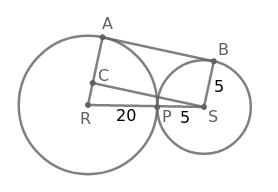AB is tangent to both the circles.

$\Rightarrow$  AB$\bot\$AR

Let SC be the line parallel to AB

$\Rightarrow$  SC$\bot\$AR and AC = BS = 5 cm and AB = SC

Radius of bigger circle AR = 20 cm

$\Rightarrow$  AC + CR = 20

$\Rightarrow$  5 + CR = 20

$\Rightarrow$  CR = 15 cm

Since circles touch externally as shown in figure

RS = RP + PS = 20 + 5 = 25 cm

In $\triangle$RCS,

CR$^2$ + SC$^2$ = RS$^2$

$\Rightarrow$  15$^2$ + SC$^2$ = 25$^2$

$\Rightarrow$  225 + SC$^2$ = 625

$\Rightarrow$  SC$^2$ = 400

$\Rightarrow$  SC = 20 cm

$\therefore\$Length of AB = SC = 20 cm

Hence, the correct answer is Option D

Question 15: The distance between the centres of two equal circles each of radius 4 cm is 17 cm. The length of a transverse tangent is:

a) 14 cm

b) 16 cm

c) 19 cm

d) 15 cm

Solution:

Given, distance between centres of the circles ($d$) = 17 cm

Radius of the two circles ($r_1$, $r_2$) = 4 cm

$\therefore\$Length of the tranverse commom tangent = $\sqrt{d^2-\left(r_1+r_2\right)^2}$

$=\sqrt{17^2-\left(4+4\right)^2}$

$=\sqrt{289-64}$

$=\sqrt{225}$

$=15$ cm

Hence, the correct answer is Option D

Question 16: ABC is a right angled triangle, right angled at A. A circle is inscribed in it. The lengths of two sides containing the right angle are 48 cm and 14 cm. The radius of the inscribed circle is:

a) 4 cm

b) 8 cm

c) 6 cm

d) 5 cm

Solution: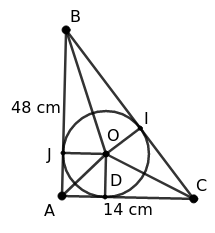Using the pythagoras theorem,

BC$^2$ = AB$^2$ + AC$^2$

$\Rightarrow$  BC$^2$ = 48$^2$ + 14$^2$

$\Rightarrow$  BC$^2$ = 2304 + 196

$\Rightarrow$  BC$^2$ = 2500

$\Rightarrow$  BC = 50 cm

Let the radius of the circle = r

$\Rightarrow$  OD = OI = OJ = r

AB, BC, AC are tangents to the circle

Area of $\triangle$ABC = Area of $\triangle$OAC + Area of $\triangle$OBC + Area of $\triangle$OAB

$\Rightarrow$  $\frac{1}{2}$ x 48 x 14 = $\frac{1}{2}$ x AC x OD + $\frac{1}{2}$ x BC x OI + $\frac{1}{2}$ x AB x OJ

$\Rightarrow$  336 = $\frac{1}{2}$ x 14 x r + $\frac{1}{2}$ x 50 x r + $\frac{1}{2}$ x 48 x r

$\Rightarrow$  336 = 7r + 25r + 24r

$\Rightarrow$  56r = 336

$\Rightarrow$  r = 6 cm

$\therefore\$Radius of the inscribed circle = 6 cm

Hence, the correct answer is Option C

Question 17: A is point at a distance 26 cm from the centre O of a circle of radius 10 cm. AP and AQ are the tangents to the circle at the point of contacts P and Q. If a tangent BC is drawn at a point R lying on the minor arc PQ to intersect AP at B and AQ at C, then the perimeter of $\triangle$ABC is:

a) 48 cm

b) 46 cm

c) 42 cm

d) 40 cm

Solution: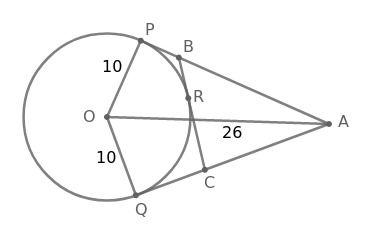Given, A is point at a distance 26 cm from the centre O

Radius of the circle = 10 cm

AP and AQ are tangents to the circle at the point of contacts P and Q

$\Rightarrow$ AP$\bot\$OP and AQ$\bot\$OQ

The length of tangents to the circle from an external point are equal.

$\Rightarrow$  AP = AQ ……………..(1)

Similarly, BP = BR and CQ = CR …….(2)

In $\triangle$OPA,

OP$^2$ + AP$^2$ = AO$^2$

$\Rightarrow$  10$^2$ + AP$^2$ = 26$^2$

$\Rightarrow$  100 + AP$^2$ = 676

$\Rightarrow$  AP$^2$ = 576

$\Rightarrow$  AP = 24 cm

Perimeter of $\triangle$ABC = AB + BC + AC

= AB + BR + CR + AC

= AB + BP + CQ + AC [From (2)]

= AP + AQ

= AP + AP [From (1)]

= 2AP

= 2(24)

= 48 cm

Hence, the correct answer is Option A

Question 18: A, B, C are three points so that AB = 4 cm, BC = 6 cm and AC = 10 cm. The number of circles passing through the points A, B, C is:

a) 1

b) 3

c) 0

d) 2

Solution: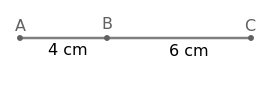AB + BC = 4 + 6 = 10 cm

AB + BC = AC

$\Rightarrow$  A, B, C are collinear points

$\therefore\$No circle can be formed using three collinear points

Hence, the correct answer is Option C

Question 19: In a circle centred at O, AB is a chord and C is any point on AB, such that OC is perpendicular to AB. If the length of the chord is 16 cm and OC = 6 cm, the radius of circle is:

a) 8 cm

b) 12 cm

c) 10 cm

d) 6 cm

Solution: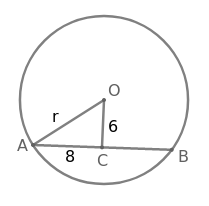Let the radius of the circle = r

Given, AB = 16 cm, OC = 6 cm

OC is perpendicular to the chord AB.

In a circle, perpendicular from centre of the circle to the chord bisects the chord.

$\Rightarrow$ AC = BC = 8 cm

In $\triangle$OAC,

AC$^2$ + OC$^2$ = OA$^2$

$\Rightarrow$ 8$^2$ + 6$^2$ = r$^2$

$\Rightarrow$ 64 + 36 = r$^2$

$\Rightarrow$ r$^2$ = 100

$\Rightarrow$  r = 10 cm

$\therefore\$Radius of the circle = 10 cm

Hence, the correct answer is Option C

Question 20: If the angle between two radii of a circle be 130$^\circ$, then the angle between the tangents at the end of these radii (in degrees)is:

a) $50^\circ$

b) $90^\circ$

c) $40^\circ$

d) $70^\circ$

Solution: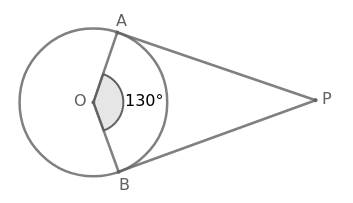Angle between the two radii OA and OB = 130$^\circ$

PA and PB are tangents at A and B to the circle

$\Rightarrow$  $\angle$OAP = 90$^\circ$ and $\angle$OBP = 90$^\circ$

$\angle$OAP + $\angle$AOB + $\angle$OBP + $\angle$APB = 360$^\circ$
$\Rightarrow$ 90$^\circ$ +  130$^\circ$ + 90$^\circ$ + $\angle$APB = 360$^\circ$
$\Rightarrow$ 310$^\circ$ + $\angle$APB = 360$^\circ$
$\Rightarrow$ $\angle$APB = 50$^\circ$
$\therefore\$Angle between the tangents = $\angle$APB = 50$^\circ$